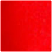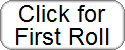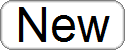# How to Play Poker Dice

## aka Yahtzee (TM)hold hold hold hold holdH A D 2 K N O W . o r g   P O K E R D I C E

Poker dice is played according rules similar to that of card poker. In poker dice, the object is the attain a combination of dice that ranks higher than your opponents' combinations. You get three chances to roll the dice, and you may hold any number of dice when you roll.

Yahtzee (TM) is a trademarked version of poker dice sold by Hasbro. In Yahtzee, the object is to attain different combinations and earn points for each achievement. The player with the highest score wins.

Below we explain the rules for each game below and explain the probabilities of rolling certain combinations. You can also try your luck with the poker dice/Yahtzee game above.

## Poker Dice Rules

In traditional poker dice, each player has three opportunities to roll five dice. After the first roll, you may hold some dice and roll the remaining dice. After the second roll, you may hold more dice or release previously held dice before rolling for the last time. After the third roll, the dice are examined. There are eight possible outcomes shown in the table below. The right column shows the probability of attaining each outcome in a single throw of the dice.
 Rank Combination Name Probability Highest Five of a Kind 1/1296 = 0.0007716 2 Four of a Kind 25/1296 = 0.01929 3 Full House 25/648 = 0.03858 4 Straight 5/162 = 0.03086 5 Three of a Kind 25/162 = 0.1543 6 Two Pairs 25/108 = 0.2315 7 One Pair 25/54 = 0.463 Lowest High Card (High Die) 5/81 = 0.06173

Note that even though a straight is harder to roll than a full house, full house outranks straight. Also note that the probability of having nothing but a high card is quite low, only 6%.

## Yahtzee Rules and scoring

In Yahtzee, you score points for making some of the poker dice combinations above, plus other combinations not listed above. In a typical game, each player has 13 turns to make each combination, in any order. As in poker dice, players are allowed three rolls per turn. Here are the 13 scoreable combinations:
 Name Points Name Points Ones sum of the 1's Chance sum of the dice Twos sum of the 2's Three of a Kind sum of the dice Threes sum of the 3's Four of a Kind sum of the dice Fours sum of the 4's Full House 25 Fives sum of the 5's Small Straight 30 Sixes sum of the 6's Large Straight 40 Bonus 35 Yahtzee 50

Explanations:
• Small Straight: A straight on four dice; 1234, 2345, or 3456. The probability of rolling this combo is 10/81 = 0.1235.
• Large Straight: A straight on all five dice; 12345 or 23456.
• Yahtzee: Five of a kind.
• Chance: Simply the sum of the dice.
• Bonus: When a player attains a subscore of at least 63 on the ones, twos,..., sixes, then the player gets an additional 35 points. In order to earn a score of at least 63 on the first six categories, one should roll at least three ones, three twos,..., three sixes.
Additionally, there is the chance of earning a Yahtzee Bonus. This is worth 100 points if the player makes more than one yahtzee in a game. If a player receives one or more Yahtzee bonuses, the player may require more than 13 turns to complete the game.